﻿ 广义度量空间问题成立的可能性

# 广义度量空间问题成立的可能性The Possibility of the Problem on Generalized Metric Space

Abstract: This paper tries to prove the equivalence of stratifiable spaces in the problem on generalized metric space. The definitions and properties of finite basis and regularity in metric space are studied. The regular space with   local finite basis is obtained. In addition, the method of building space is obtained by using the Nagata-Smirnov Metrization Theorem to weaken the   local finite basis to the closure keep basis. A method of constructing a topological space and establishing a continuous mapping is used here. The space M1 is equal to the space M3. In this way, three kinds of spatial equivalent problems in generalized metric space are solved.

1. 引言

2. M1与M3等价的证明过程

2.1. 引入紧缩映射 $\Phi$$\Phi$ 具有如下性质

1) 若 $A\cap B=\varnothing$，显然 ${H}_{A}\cap {H}_{B}=\varnothing$

2) 若 $A\cap B\ne \varnothing$，必有 ${H}_{A}\cap {H}_{B}=\varnothing$

1)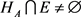${H}_{B}\cap E\ne \varnothing$

2)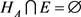${H}_{B}\cap E\ne \varnothing$

3) ${H}_{A}\cap E\ne \varnothing$${H}_{B}\cap E=\varnothing$

4) ${H}_{A}\cap E=\varnothing$${H}_{B}\cap E=\varnothing$

2.2. 紧缩映射的性质的进一步讨论

3. 层空间对应关系的讨论

3.1. 证明 ${H}_{{M}_{1}}$ 空间是 ${M}_{1}$ 空间

${T}_{11}=\underset{i=1}{\overset{\infty }{\cup }}{W}_{i11}\cup {A}_{1},\cdots ,{T}_{1n}=\underset{i=1}{\overset{\infty }{\cup }}{W}_{i1n}\cup {A}_{1};\cdots ;{T}_{nn}=\underset{i=1}{\overset{\infty }{\cup }}{W}_{inn}\cup {A}_{n}$.

$\underset{i,k}{\overset{\infty }{\cap }}{T}_{ik}={A}_{k}$ 时， $\left(\underset{i=1}{\overset{\infty }{\cap }}{T}_{i1}\right)\cup \left(\underset{i=1}{\overset{\infty }{\cap }}{T}_{i2}\right)\cup \cdots ={A}_{1}\cup {A}_{2},\cdots$ 显然成立。

${T}_{it}=\left\{{T}_{it}-\left({Q}_{t}\cup {A}_{t}\right)\right\}\cup \left({Q}_{t}\cup {A}_{t}\right)=\underset{g=1}{\overset{\infty }{\cup }}{K}_{g}\cup {A}_{t}$.

$\left(\underset{i=1}{\overset{\infty }{\cap }}{T}_{i1}\right)\cup \left(\underset{i=1}{\overset{\infty }{\cap }}{T}_{i2}\right)\cup \cdots =\left(\underset{e=1}{\overset{\infty }{\cup }}{Q}_{e}\right)\cup \left(\underset{e=1}{\overset{\infty }{\cup }}{A}_{e}\right)=\underset{j=1}{\overset{\infty }{\cap }}{N}_{j}=\underset{\mu =1}{\overset{\infty }{\cup }}{A}_{\mu }\cup P$

${N}_{j}\cap {T}_{i1}={A}_{1},{N}_{j}\cap {T}_{i2}={A}_{2},\cdots$，其中$⇒\underset{i,j,\Sigma }{\overset{\infty }{\cup }}\left({N}_{j}\cap {T}_{i\Sigma }\right)=\underset{\varphi =1}{\overset{\infty }{\cup }}{A}_{\varphi }⇒\left(\underset{j=1}{\overset{\infty }{\cup }}{N}_{j}\right)\cap \left(\underset{i,\Sigma }{\overset{\infty }{\cup }}{T}_{i\Sigma }\right)=\underset{\varphi =1}{\overset{\infty }{\cup }}{A}_{\varphi }$.

$\left(\underset{i=1}{\overset{\infty }{\cap }}{T}_{i1}\right)\cup \left(\underset{i=1}{\overset{\infty }{\cap }}{T}_{i2}\right)\cup \cdots =\left(\underset{e=1}{\overset{\infty }{\cup }}{Q}_{e}\right)\cup \left(\underset{e=1}{\overset{\infty }{\cup }}{A}_{e}\right)$ ,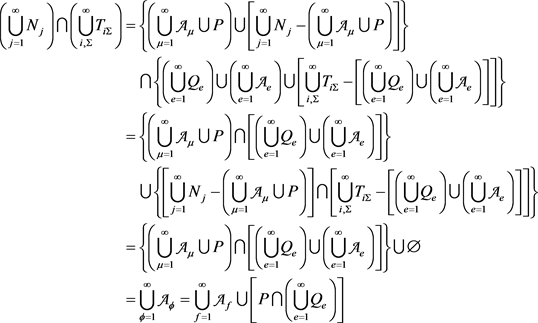, (1)

$\left\{{P}_{i}-\left(\underset{\varphi =1}{\overset{\infty }{\cup }}{A}_{\varphi }\right)\right\}\cap \left\{\left(\underset{e=1}{\overset{\infty }{\cup }}{Q}_{e}\right)-\left(\underset{\varphi =1}{\overset{\infty }{\cup }}{A}_{\varphi }\right)\right\}\subset \underset{\varphi =1}{\overset{\infty }{\cup }}{A}_{\varphi }$，同样地，也可能出现例如 $\underset{\varphi =1}{\overset{\infty }{\cup }}{A}_{\varphi }\subset P$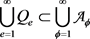，如此则总有 $\underset{f=1}{\overset{\infty }{\cup }}{A}_{f}\cup \left\{P-\left(\underset{\varphi =1}{\overset{\infty }{\cup }}{A}_{\varphi }\right)\right\}=\underset{\varphi =1}{\overset{\infty }{\cup }}{A}_{\varphi }⇒P-\left(\underset{\varphi =1}{\overset{\infty }{\cup }}{A}_{\varphi }\right)=\varnothing ⇒P\subset \underset{\varphi =1}{\overset{\infty }{\cup }}{A}_{\varphi }$

${N}_{j}\cap {T}_{i1}={A}_{1}\cup {B}_{1},{N}_{j}\cap {T}_{i2}={A}_{2}\cup {B}_{2},\cdots$ 时，可由(1)式得到

$\left(\underset{j=1}{\overset{\infty }{\cup }}{N}_{j}\right)\cap \left(\underset{i,\Sigma }{\overset{\infty }{\cup }}{T}_{i\Sigma }\right)=\underset{f=1}{\overset{\infty }{\cup }}{A}_{f}\cup \left[P\cap \left(\underset{e=1}{\overset{\infty }{\cup }}{Q}_{e}\right)\right]=\left(\underset{\varphi =1}{\overset{\infty }{\cup }}{A}_{\varphi }\right)\cup \left(\underset{\varphi =1}{\overset{\infty }{\cup }}{B}_{\varphi }\right)$ ,

${N}_{j}\cap {T}_{i1}={T}_{i1},{N}_{j}\cap {T}_{i2}={T}_{i2},\cdots$ 时，仍然由(1)式可得：

$\left(\underset{j=1}{\overset{\infty }{\cup }}{N}_{j}\right)\cap \left(\underset{i,\Sigma }{\overset{\infty }{\cup }}{T}_{i\Sigma }\right)=\underset{f=1}{\overset{\infty }{\cup }}{A}_{f}\cup \left[P\cap \left(\underset{e=1}{\overset{\infty }{\cup }}{Q}_{e}\right)\right]=\underset{i,\Sigma }{\overset{\infty }{\cup }}{T}_{i\Sigma }$ ;

$P\cap \left(\underset{e=1}{\overset{\infty }{\cup }}{Q}_{e}\right)=\varnothing$ 时，即 $\underset{f=1}{\overset{\infty }{\cup }}{A}_{f}\cup \varnothing =\underset{f=1}{\overset{\infty }{\cup }}{A}_{f}=\underset{i,\Sigma }{\overset{\infty }{\cup }}{T}_{i\Sigma }$ 与之前所假设情况的其中之一符合，所以显然成立；当 $P\cap \left(\underset{e=1}{\overset{\infty }{\cup }}{Q}_{e}\right)=Z\ne \varnothing$ 时，得 $\underset{f=1}{\overset{\infty }{\cup }}{A}_{f}\cup Z=\underset{i,\Sigma }{\overset{\infty }{\cup }}{T}_{i\Sigma }$ 也同样如此。因此不论 $P\cap \left(\underset{e=1}{\overset{\infty }{\cup }}{Q}_{e}\right)$ 的情况如何，都有 $P=\underset{e=1}{\overset{\infty }{\cup }}{Q}_{e}$ 成立。所以式子 $\left(\underset{i=1}{\overset{\infty }{\cap }}{T}_{i1}\right)\cup \left(\underset{i=1}{\overset{\infty }{\cap }}{T}_{i2}\right)\cup \cdots =\underset{j=1}{\overset{\infty }{\cap }}{N}_{j}$ 得证。所以经映射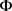作用后所得到的空间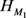的确是 ${M}_{1}$ 空间。

3.2. 证明 ${H}_{{M}_{3}}$ 空间是 ${M}_{3}$ 空间

${E}_{1}\subset {U}_{11},{U}_{21},\cdots ;\cdots ;{E}_{n}\subset {U}_{1n},{U}_{2n},\cdots$.

${M}_{3}$ 空间的定义可类似地对 ${H}_{M3}$ 就有，对于另一闭集D， ${G}_{1},{G}_{2},\cdots$ 为开集，所以可设

${E}_{1}\cup {{E}^{\prime }}_{1}=\underset{k=1}{\overset{\infty }{\cap }}{U}_{K1},{E}_{2}\cup {{E}^{\prime }}_{2}\subset \underset{K=1}{\overset{\infty }{\cap }}{U}_{K2},\cdots$.

$\begin{array}{c}C=\left({E}_{1}\cup {{E}^{\prime }}_{1}\right)\cap \left({E}_{2}\cup {{E}^{\prime }}_{2}\right)\cap \cdots =\left({E}_{1}\cap {E}_{2}\cap \cdots \right)\cup \left({{E}^{\prime }}_{1}\cap {{E}^{\prime }}_{2}\cap \cdots \right)\\ =\left(\underset{c=1}{\overset{\infty }{\cap }}{E}_{c}\right)\cup \left(\underset{d=1}{\overset{\infty }{\cap }}{{E}^{\prime }}_{d}\right)={C}^{\prime }\cup \left(\underset{d=1}{\overset{\infty }{\cap }}{{E}^{\prime }}_{d}\right)\end{array}$ ,

$D=\left({G}_{1}\cup {{G}^{\prime }}_{1}\right)\cap \left({G}_{2}\cup {{G}^{\prime }}_{2}\right)\cap \cdots =\left(\underset{a=1}{\overset{\infty }{\cap }}{G}_{a}\right)\cup \left(\underset{b=1}{\overset{\infty }{\cap }}{{G}^{\prime }}_{b}\right)={D}^{\prime }\cup \left(\underset{b=1}{\overset{\infty }{\cap }}{{G}^{\prime }}_{b}\right)$

$C=D$ 时，就有 $D-\left(\underset{b=1}{\overset{\infty }{\cap }}{{G}^{\prime }}_{b}\right)=C-\left(\underset{d=1}{\overset{\infty }{\cap }}{{E}^{\prime }}_{d}\right)$，当 $\left({E}_{i}-C\right)\cup \left(\underset{d=1}{\overset{\infty }{\cap }}{{E}^{\prime }}_{d}\right)\subset \left({G}_{j}-D\right)\cup \left(\underset{b=1}{\overset{\infty }{\cap }}{{G}^{\prime }}_{b}\right)$ 时， ${H}_{{M}_{3}}$ 是广义度量空间。

$C\subset D$ 时，设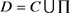，所以 $D-\left(\underset{b=1}{\overset{\infty }{\cap }}{{G}^{\prime }}_{b}\right)=\left[C-\left(\underset{d=1}{\overset{\infty }{\cap }}{{E}^{\prime }}_{d}\right)\right]\cup \prod$，所以当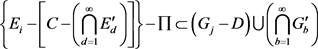$\left({E}_{i}-C\right)\cup \left(\underset{d=1}{\overset{\infty }{\cap }}{{E}^{\prime }}_{d}\right)\subset \left({G}_{j}-D\right)\cup \left(\underset{b=1}{\overset{\infty }{\cap }}{{G}^{\prime }}_{b}\right)\cup \prod$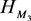是广义度量空间。

$C,D$ 为闭集， ${E}_{1},{E}_{2},\cdots$${G}_{1},{G}_{2},\cdots$ 为开集并且

${E}_{1}\subset \underset{k=1}{\overset{\infty }{\cap }}{U}_{k1},{E}_{2}\subset \underset{k=1}{\overset{\infty }{\cap }}{U}_{k2},\cdots$ , ${G}_{1}\subset \underset{\delta =1}{\overset{\infty }{\cap }}{F}_{\delta 1},{G}_{2}\subset \underset{\delta =1}{\overset{\infty }{\cap }}{F}_{\delta 2},\cdots$.

$D=\left({G}_{1}\cup {L}_{1}\right)\cap \left({G}_{2}\cup {L}_{2}\right)\cap \cdots =\left(\underset{\delta =1}{\overset{\infty }{\cap }}{G}_{\delta }\right)\cup \left(\underset{\lambda =1}{\overset{\infty }{\cap }}{L}_{\lambda }\right)$

$C=\left({E}_{1}\cup {K}_{1}\right)\cap \left({E}_{2}\cup {K}_{2}\right)\cap \cdots =\left(\underset{k=1}{\overset{\infty }{\cap }}{E}_{k}\right)\cup \left(\underset{\mu =1}{\overset{\infty }{\cap }}{K}_{\mu }\right)$.

$D=\left(\underset{k=1}{\overset{\infty }{\cap }}{E}_{k}\right)\cup \left(\underset{s=1}{\overset{\infty }{\cap }}{J}_{s}\right)\cup \left(\underset{\lambda =1}{\overset{\infty }{\cap }}{L}_{\lambda }\right)=\left\{C-\left(\underset{\mu =1}{\overset{\infty }{\cap }}{K}_{\mu }\right)\right\}\cup \left(\underset{s=1}{\overset{\infty }{\cap }}{J}_{s}\right)\cup \left(\underset{\lambda =1}{\overset{\infty }{\cap }}{L}_{\lambda }\right)$.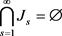$\underset{\mu =1}{\overset{\infty }{\cap }}{K}_{\mu }\subset \underset{\lambda =1}{\overset{\infty }{\cap }}{L}_{\lambda }$ 时有 $C\subset D$ 成立。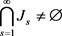时有 $C\subset D$ 成立。

3.3. 讨论 ${H}_{{M}_{1}}\sim {H}_{{M}_{3}}$ 的成立性

$C,E\subset A\subset {H}_{{M}_{1}}$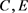都是闭集， $A$ 为保持基，若 $E=\left(\underset{t=1}{\overset{\infty }{\cup }}{W}_{t}\right)\cap C$，其中 ${W}_{1},{W}_{2},\cdots$$C,E$ 都是闭集，且E满足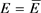，令 $C={J}_{1}\cup {J}_{2}\cup \cdots$。因为由保持基的条件就有

$\left(\underset{i=1}{\overset{\infty }{\cap }}{R}_{i1}\right)\cup \left(\underset{i=1}{\overset{\infty }{\cap }}{R}_{i2}\right)\cup \cdots =\underset{j=1}{\overset{\infty }{\cap }}{S}_{j}$ (2)

$\left\{\left(\underset{i=1}{\overset{\infty }{\cap }}{R}_{i1}\right)\cup \left(\underset{i=1}{\overset{\infty }{\cap }}{R}_{i2}\right)\cup \cdots \right\}\cap \left\{\left(\underset{i=1}{\overset{\infty }{\cap }}{K}_{i1}\right)\cup \left(\underset{i=1}{\overset{\infty }{\cap }}{K}_{i2}\right)\cup \cdots \right\}=\left(\underset{j=1}{\overset{\infty }{\cap }}{S}_{j}\right)\cap \left(\underset{j=1}{\overset{\infty }{\cap }}{L}_{j}\right)=\underset{\theta =1}{\overset{\infty }{\cap }}\left\{\left(\underset{i=1}{\overset{\infty }{\cap }}{R}_{i\theta }\right)\cap \left(\underset{i=1}{\overset{\infty }{\cap }}{K}_{i\theta }\right)\right\}$

${J}_{1}\cup {Z}_{1}=\underset{i=1}{\overset{\infty }{\cap }}{K}_{i1},{J}_{2}\cup {Z}_{2}=\underset{i=1}{\overset{\infty }{\cap }}{K}_{i2},\cdots$ ,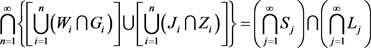. (4)

$\underset{i=1}{\overset{\infty }{\cap }}{T}_{i1}={P}_{1}\cup {Q}_{1},\underset{i=1}{\overset{\infty }{\cap }}{T}_{i2}={P}_{2}\cup {Q}_{2},\cdots$

$\left(\underset{i=1}{\overset{\infty }{\cap }}{T}_{i1}\right)\cap \left(\underset{i=1}{\overset{\infty }{\cap }}{T}_{i2}\right)\cap \cdots =E$ ,

$C=\left(\underset{\epsilon =1}{\overset{\infty }{\cap }}{I}_{\epsilon 1}\right)\cap \left(\underset{\epsilon =1}{\overset{\infty }{\cap }}{I}_{\epsilon 2}\right)\cap \cdots =\left(\underset{c=1}{\overset{\infty }{\cap }}{F}_{c}\right)\cup \left(\underset{d=1}{\overset{\infty }{\cap }}{{F}^{\prime }}_{d}\right)$，所以 $\left(\underset{c=1}{\overset{\infty }{\cap }}{F}_{c}\right)\cup \left(\underset{d=1}{\overset{\infty }{\cap }}{{F}^{\prime }}_{d}\right)\cup Q=\left(\underset{a=1}{\overset{\infty }{\cap }}{E}_{a}\right)\cup \left(\underset{b=1}{\overset{\infty }{\cap }}{{E}^{\prime }}_{b}\right)$

$C,D\subset {H}_{{M}_{3}}$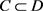$C,D$ 都是闭集，若 $C=D=\underset{t=1}{\overset{\infty }{\cup }}{V}_{t}$，且有一闭集可表示为开集的闭包的交，即有 $C=\underset{k,l}{\overset{\infty }{\cap }}{U}_{kl}=D=\underset{t=1}{\overset{\infty }{\cup }}{V}_{t}$。当 ${V}_{1}=\underset{i=1}{\overset{\infty }{\cap }}{E}_{i1},{V}_{2}=\underset{i=1}{\overset{\infty }{\cap }}{E}_{i2},\cdots$ 时， ${E}_{i1}$${V}_{1}={W}_{11}\cup {W}_{21}\cup \cdots$${W}_{i1}$ 的闭包，……， ${E}_{in}$${V}_{n}={W}_{1n}\cup {W}_{2n}\cup \cdots$${W}_{in}$ 的闭包，……。所以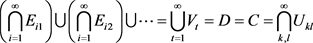,

$C\subset D$，设 $D=C\cup Q=\underset{t=1}{\overset{\infty }{\cup }}{V}_{t}\cup Q$$C,D$ 是闭集，且有 $D=\underset{\delta ,\theta }{\overset{\infty }{\cap }}{G}_{\delta \theta }$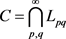。因为 $G\left(n,C\right)\subset G\left(n,D\right)$，所以有 ${M}_{1}\subset \underset{\delta =1}{\overset{\infty }{\cap }}{G}_{\delta 1},{M}_{2}\subset \underset{\delta =1}{\overset{\infty }{\cap }}{G}_{\delta 2},\cdots$${N}_{1}\subset \underset{p=1}{\overset{\infty }{\cap }}{L}_{p1},{N}_{2}\subset \underset{p=1}{\overset{\infty }{\cap }}{L}_{p2},\cdots$ 所以设 ${N}_{i}\cup P={M}_{j}$ 其中，同样也有 ${M}_{1}\cup {{M}^{\prime }}_{1}=\underset{\delta =1}{\overset{\infty }{\cap }}{G}_{\delta 1},{M}_{2}\cup {{M}^{\prime }}_{2}=\underset{\delta =1}{\overset{\infty }{\cap }}{G}_{\delta 2},\cdots$${N}_{1}\cup {{N}^{\prime }}_{1}=\underset{p=1}{\overset{\infty }{\cap }}{L}_{p1},{N}_{2}\cup {{N}^{\prime }}_{2}=\underset{p=1}{\overset{\infty }{\cap }}{L}_{p2},\cdots$ 所以

$D=\underset{\delta ,\theta }{\overset{\infty }{\cap }}{G}_{\delta \theta }=\left({M}_{1}\cup {{M}^{\prime }}_{1}\right)\cap \left({M}_{2}\cup {{M}^{\prime }}_{2}\right)\cap \cdots =\left(\underset{a=1}{\overset{\infty }{\cap }}{M}_{a}\right)\cup \left(\underset{b=1}{\overset{\infty }{\cap }}{{M}^{\prime }}_{b}\right)=\left(\underset{a=1}{\overset{\infty }{\cap }}{N}_{a}\right)\cup \left(\underset{\epsilon =1}{\overset{\infty }{\cap }}{P}_{\epsilon }\right)\cup \left(\underset{b=1}{\overset{\infty }{\cap }}{{M}^{\prime }}_{b}\right)$

$C=\underset{p,q}{\overset{\infty }{\cap }}{L}_{pq}=\left({N}_{1}\cup {{N}^{\prime }}_{1}\right)\cap \left({N}_{2}\cup {{N}^{\prime }}_{2}\right)\cap \cdots =\left(\underset{c=1}{\overset{\infty }{\cap }}{N}_{c}\right)\cup \left(\underset{d=1}{\overset{\infty }{\cap }}{{N}^{\prime }}_{d}\right)$

$Q=D-C=\left\{\left(\underset{\epsilon =1}{\overset{\infty }{\cap }}{P}_{\epsilon }\right)\cup \left(\underset{b=1}{\overset{\infty }{\cap }}{{M}^{\prime }}_{b}\right)\right\}-\left(\underset{d=1}{\overset{\infty }{\cap }}{{N}^{\prime }}_{d}\right)\ne \varnothing$.

4. 总结

 “10000个科学难题”数学编委会. 10000个科学难题•数学卷[M]. 北京: 科学出版社, 2009: 211-212.

 James R. Munkres. 拓扑学[M]. 北京: 机械工业出版社, 2006.

 儿玉之宏, 永见启应. 拓扑空间论[M]. 北京: 科学出版社, 1984.

 高国士. 拓扑空间论[M]. 北京: 科学出版社, 2000.

 Anderson, R.D., et al. (2002) Recent Progress in General Topology. North-Holland, Amsterdam, 545-575.

 Gartside, P.M. and Reznichenko, E.A. (2000) Near Metric Properties of Function Spaces. Fundamenta Mathematicae, 164, 97-114.

Top